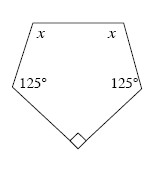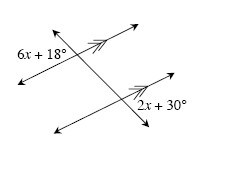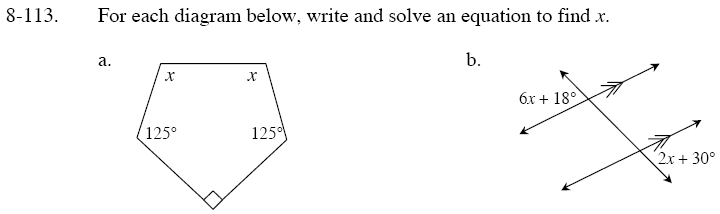Home > GC > Chapter 8 > Lesson 8.3.3 > Problem8-113

8-113.
1. For each diagram below, write and solve an equation to find x. Homework Help ✎

1.2.The sum of the interior angles = 180°(n − 2)

Subtract the value of the known angles (125°, 125°, and 90°) from the sum of the interior angles you found in step 1. Afterwards, divide by 2 to solve for x.

Note that 6x + 18° and the angle across from it are vertical angles, while that same angle and 2x + 30° are corresponding angles.
What does that mean about 6x + 18° and 2x + 30°?

x = 3